# Team:UCSF/ModelingModeling: Decision Making Circuit

The primary goal of the modeling portion for the synthetic circuit project is to create a model that will help us figure out the right parameters, given our assumptions, which will generate the desired result, a Decision Making Circuit (Figure 1). In other words, we want a circuit that exhibits behavior A given a certain input and behavior B when the input is changed. A computer model can help us rapidly prototype by providing an environment where we can test out different promoters and repression strengths in the computer before we spend the time building and testing strains in the lab. The circuit is designed to produce different outputs according to different levels of inducer by utilizing the CRISPRi system. In lower concentrations of inducer, the guide RNA (gRNA) will be made to repress RFP. In higher concentrations of inducer, another gRNA will be made to repress GFP. The circuit should express GFP at lower inducer concentrations and RFP at high inducer concentrations, as shown in Figure 1 below.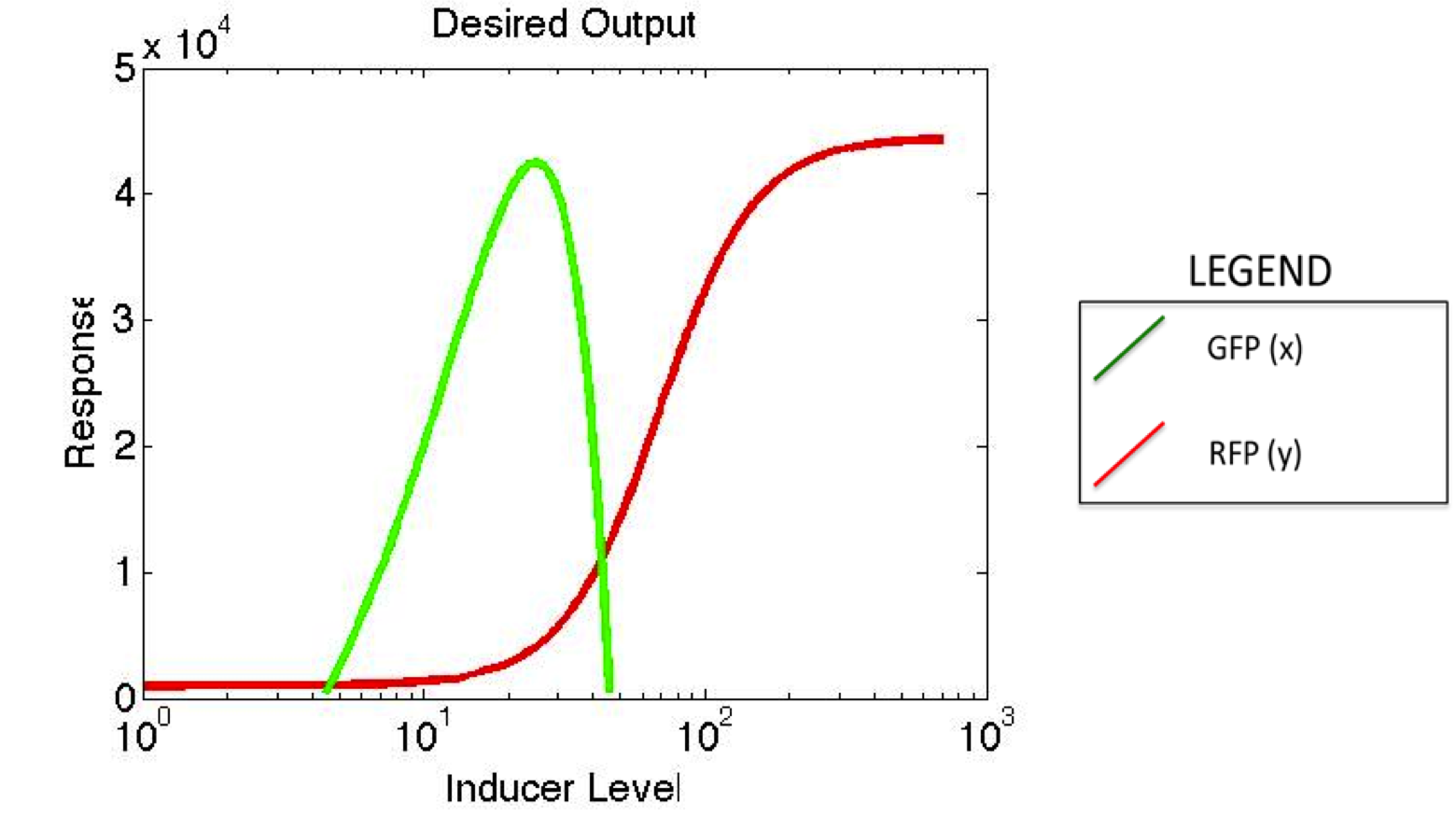If we can get this result from our model, then it would help us figure out how to change our parameters in order to generate the desired behavior.
The first step in modeling our system is to come up with a way to represent our synthetic circuit mathematically. It’s essentially the same diagram as the one shown on the synthetic circuit page, and we represent each component in the system with a different variable.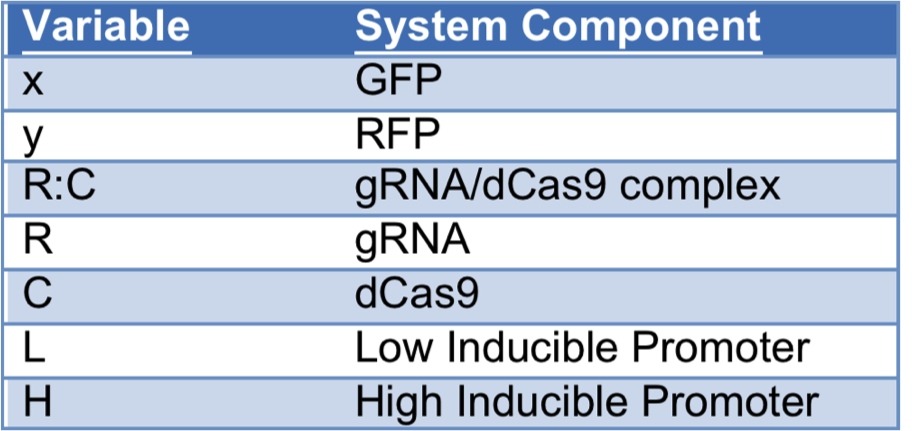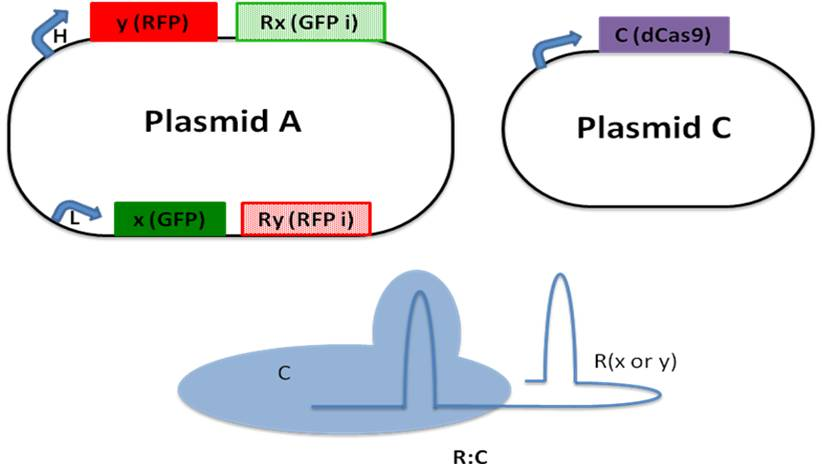ASSUMPTIONS: While creating the model for our system, we made five assumptions in order to simplify some of the aspects of the model:
2) protein production is based on a hill function and also depends on inducer concentration;
3) repression is governed by a hill function and depends on the concentration of dCas9 and gRNA complex;
4) that the binding and unbinding of dCas9 and gRNA complex happens much faster than the production/degradation of gRNA and fluorescent proteins (the complex is at Quasi Steady State).
5) everything diffuses quickly throughout the cell so that our differential equations depends on the concentration at any given time.

EQUATIONS

Given these assumptions we have the following equations for the system:
Fluorescent proteins: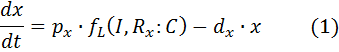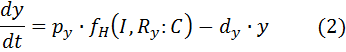In these equations, the amount of fluorescent protein depends on a production term and a degradation term. The parameters for the degradation terms are:

dx – rate of degradation of GFP based on the “low” function
dy – rate of degradation of RFP based on the “high” function

The production term consists of a function that relates inducer and repressor complex to mRNA production rate and conversion factor that relates mRNA production to protein production rate.

px – conversion factor (mRNA/protein) for x (GFP)
py – conversion factor (mRNA/protein) for y (RFP)
The mRNA production functions depend on the amount of inducer and the amount of repressor complex as follows: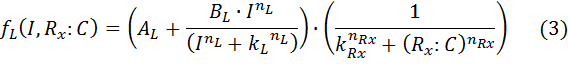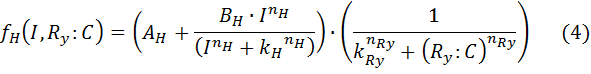There is one function for the low sensitivity promoter (fL) and another for the high sensitivity promoter (fH) . The other parameters for the production functions are:
A – basal expression level of promoter
B – maximal expression level of promoter
k – Activation coefficient for low/high promoter
kR – half maximal effective concentration of R:C
n – hill coefficient for induction.
nR – hill coefficient for repression
I – Inducer concentration
R:C – repressor/dCas9 complex.

The subscripts indicate whether the parameter is a property of the low or high sensitivity promoter (L or H) or related to protein x or y. Now we have to figure out what the equations for the gRNA/Repressor complexes are (Rx:C and Ry:C). The binding and unbinding of the gRNA and dCas9 complex is represented by the following chemical reactions: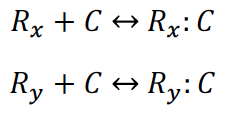Given these chemical reactions, we can write the following equations for the gRNA/dCas9 Complex: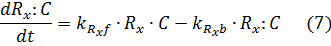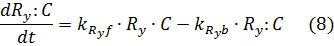Where KRxf represents the forward reaction rate for reaction (5) and KRxb represents the reverse reaction rate. The equations for the gRNA/dCas9 complex depend on the rate at which the gRNA complex with dCas9 and also the rate at which the complex breaks apart. As mentioned earlier, we made an assumption that the binding and unbinding of the gRNA/dCas9 complex happens much quicker than the production and degradation of gRNAs and fluorescent proteins. Since the binding and unbinding happen very quickly, we evaluate the equations above at steady state (equal to zero) for the given values of the other parameters.

Under that assumption (setting equations (7) and (8) to zero – known as the quasi steady state assumption), we can solve for the complex in terms of the unbound repressor concentrations: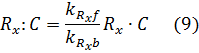Where the amount of dCas9 available in the system is given by: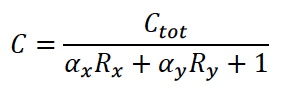The available amount of dCas9 depends on the amount of the two different complexes and also the amount of free dCas9. It has the following parameters: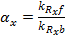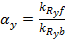The equations for the gRNAs depend on the amount of the gRNAs that is produced, the degradation rate, and also the rate at which the gRNA complexes with dCas9. With the quasi-steady state assumption, the terms for complexing with dCAS9 drop out and the final equations for the gRNAs are similar to equations (1) and (2) for the fluorescent proteins: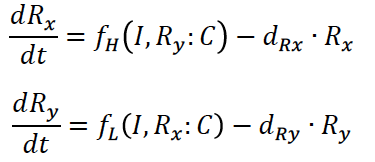PARAMETERS

This model has many parameters, so in order for it to be more useful, we need to reduce the number of parameters that are undetermined. To accomplish this, we gathered some values from literature and also did experiments to find other parameters (Table1).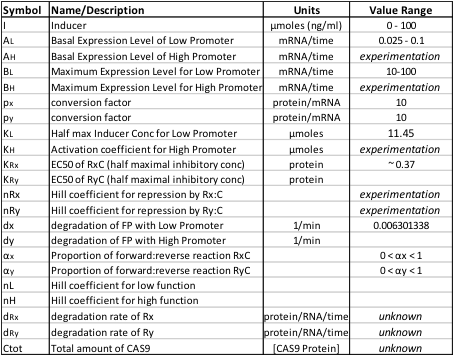How did we fit parameters? A few parameters are properties of the promoters (A, B, k, n). By determining the dosage response of a promoter to inducer we are able to fit those parameters using a curve fitting tool to get the following values.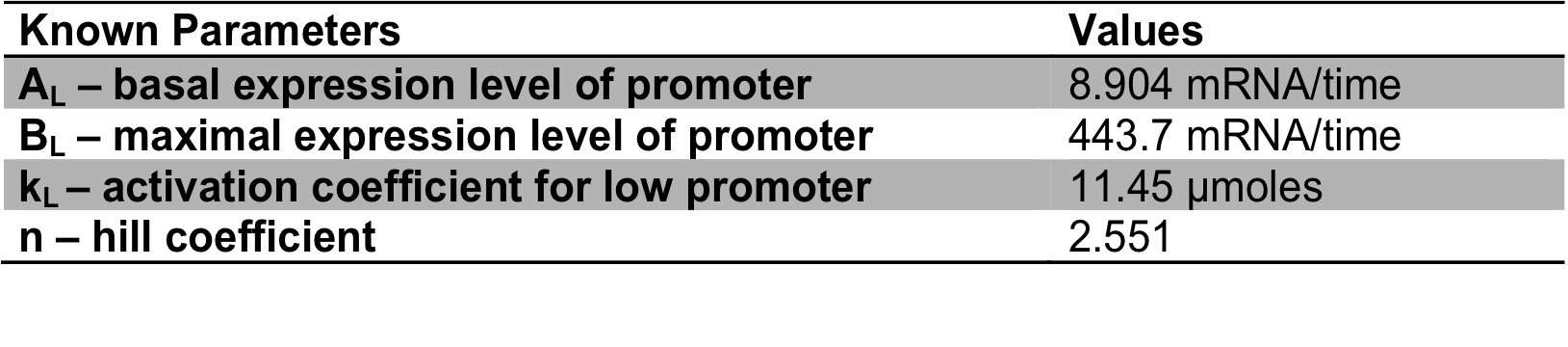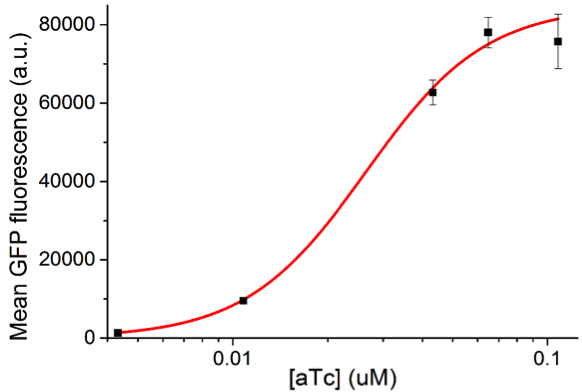How is the hill function affected by the amount of gRNA/dCas9 complex? The previous plot helped us determine a few parameters, but there are still a few parameters we have no values for from experimental data or from literature. The following plots show how the parameter for amounts of the gRNA/dCas9 complex affects the behavior of the model.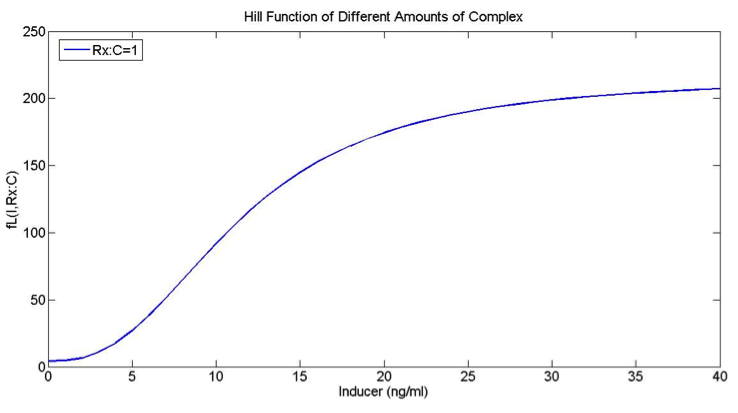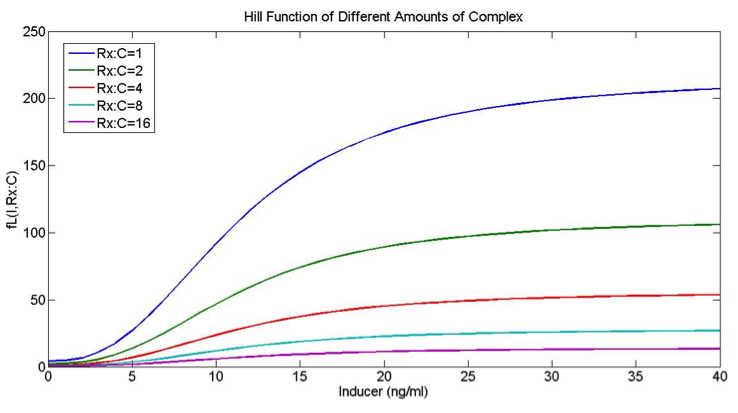From given values for inducer concentrations and amounts of complex, we can calculate the amount of fluorescent protein that should be present. Our model can help us design an experiment that helps us calculate parameters that are still unknown.

How does the model look with our actual “low” and “high” promoters?
If the only change in the low and high functions (FH and FL) is the K values (which determine the sensitivity of the promoters), then we don’t get our desired behavior. However, there are other parameters that might give us the desired behavior for the low and high promoters.
If we set BL to 443.7 and BH to 443.7*1.25, and if we set the half max values to kL = 11.45 and kH=17, the promoters have the following profile: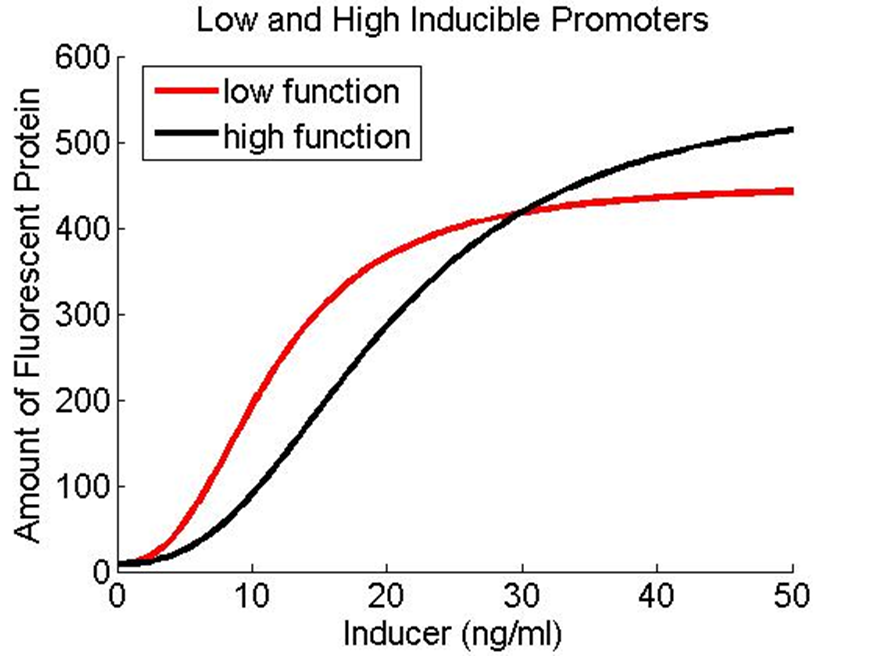And they generate the following behavior in the full model: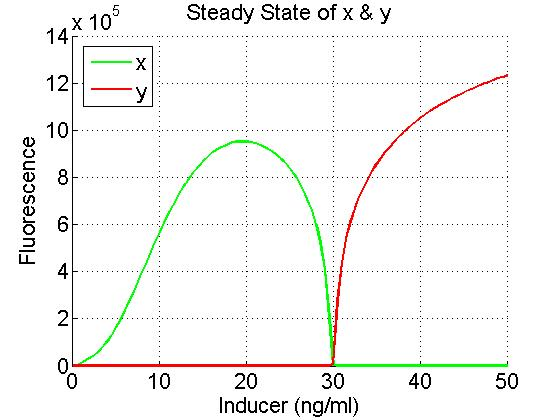Thus, based on our model, if certain conditions are met, our synthetic circuit will work as expected.

How does the system change when the hill coefficient is manipulated? In this first plot, the hill coefficients for both the low and the high function are the same number: 2.551. This number is the one we determined from our experimental data.

nL = 2.551
nH = 2.551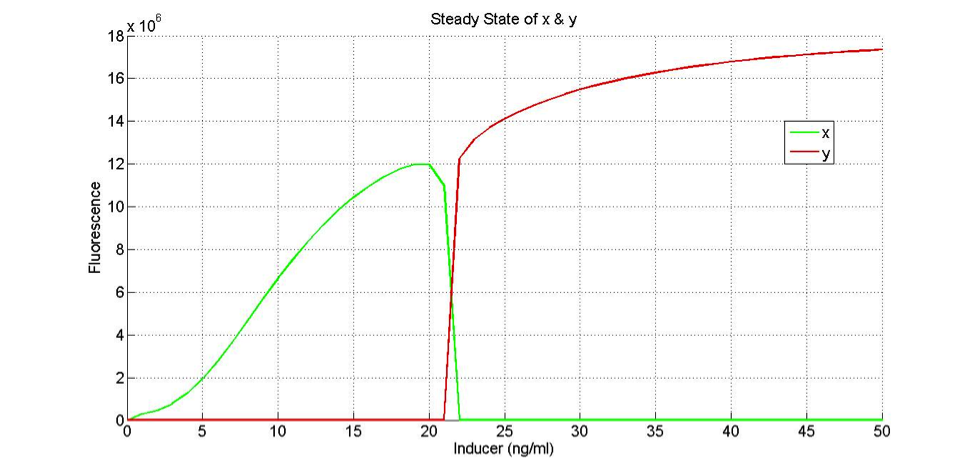We can see that the switch from GFP to RFP is relatively sharp, and that RFP seems to be expressed in higher concentrations of inducer, while GFP is being expressed in lower concentrations. In this next plot, the only thing that changed was the hill coefficient for the high function. In the first plot, it was 2.551, in the second plot, it is 1.551.

nL = 2.551
nH = 1.551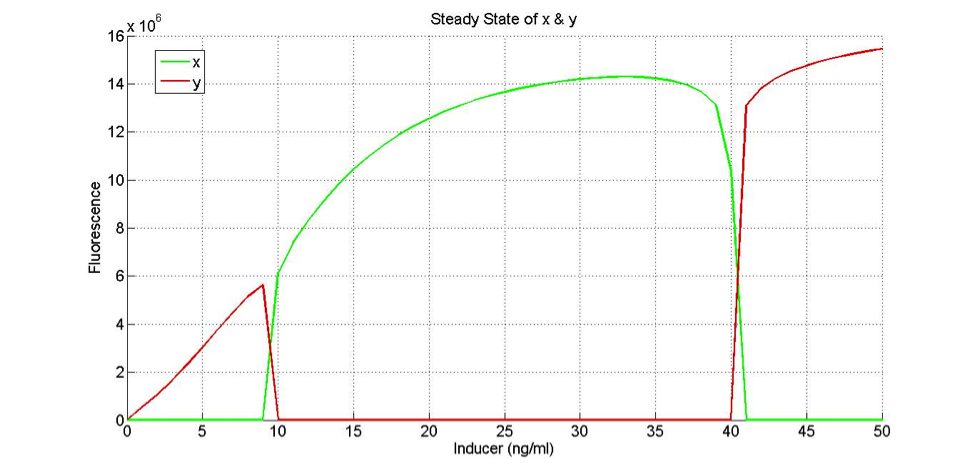It is similar to the first graph in that the switch between GFP and RFP is sharp. However, RFP is being expressed in both low and high concentrations of inducer, while GFP is being expressed in medium concentrations.

Based on our model, if certain conditions are met, our synthetic circuit will work well and work as expected.